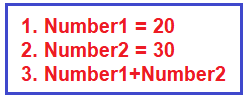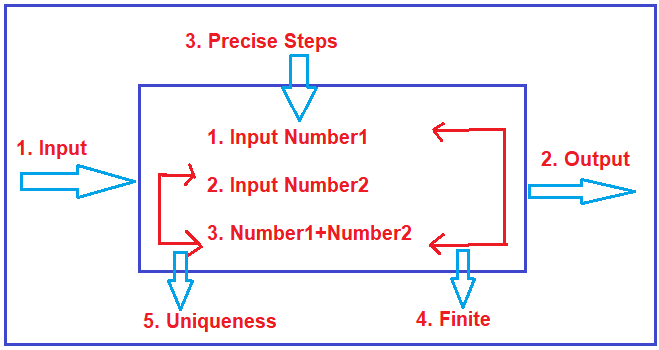# What is Algorithm

## What is an Algorithm?

In this article, I am going to discuss what is an algorithm with examples. Please read our previous article where we discussed Time and Space Complexity in detail. At the end of this article, you will learn what is an algorithm and what are the characteristics and properties of a good algorithm.

##### What is an Algorithm?

An algorithm is a step-by-step procedure or formula to solve a problem. And the step-by-step procedure is unambiguous. For example, you have a problem with sorting or you have a problem with doing some mathematical calculations. So, for that, there are time-tested algorithms wherein the precise steps, the precise input, and the precise output are defined. And if you follow that algorithm, you will be able to solve that problem.

For example, if you want to add two numbers, the steps are crystal clear and there is no ambiguity. First, take number 1, then take number 3 and then add number1 and number 2 as shown in the below image. So, algorithms mean All go in a rhythm.##### Algorithms Characteristics:

Every code which we see in a project or every code which we write in a project does not classify as an algorithm. For example, if we write an insert operation or update operation or delete operation, it does not classify as an algorithm. To classify something like an algorithm, it has to satisfy five important characteristics.First, there should be well-defined input and well-defined output. So, for an algorithm, the precise input and output should be crystal clear.

Next, the steps of the algorithms are precise, unambiguous, and to the points. For example, to add two numbers, the step should be first taking input number1 and then take input number2 and finally add number1 with number2. So, the steps for the algorithm are precise clear and it is finite.

Finite means this algorithm will start and will end after certain steps. It cannot just go in an infinite loop. Uniqueness means the output of one step will go as an input into the next step. For example, the output of step1 i.e. Numbet1 must be used somewhere down below.

##### Properties of a good algorithm
1. Input and output should be clearly defined.
2. Each step in the algorithm should be clear and unambiguous.
3. Algorithms should be most effective among many different ways to solve a problem.

Note: The algorithm should be written in such a way that it can be used in different programming languages. No coding is allowed.